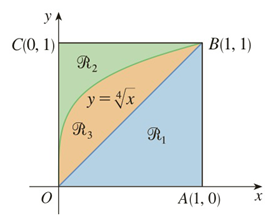Chapter 5.2, Problem 26E

Chapter
Section
Textbook Problem

# Refer to the figure and find the volume generated by rotating the given region about the specified line.ℛ 2 about BC

To determine

To find:

The volume generated by rotating the region R2 about the line  BC by using the figure.

Explanation

1)  Concept:

i. If the cross section is a disc and the radius of the disc is in terms of x  or y, then the area A=π radius2

ii. The volume of  the solid revolution about the y-axis is

V= abA(y)dy

2) Given:

Figure:

3) Calculation:

Rotating R2 about BC that is the line y=1, the cross section is perpendicular to the x-axis and it is a disc with the radius r=1-x4. Because it is the distance from the curve y= x4 to the axis of the rotation line BC (y=1)

The solid lies between x=0 and  x=1

### Still sussing out bartleby?

Check out a sample textbook solution.

See a sample solution

#### The Solution to Your Study Problems

Bartleby provides explanations to thousands of textbook problems written by our experts, many with advanced degrees!

Get Started

#### Find the limit. 44. limni=1n1n[(in)3+1]

Single Variable Calculus: Early Transcendentals, Volume I

#### let f(x) = x 1, g(x) = x+1, and h(x) = 2x3 1. Find the rule for each function. 15. fgh

Applied Calculus for the Managerial, Life, and Social Sciences: A Brief Approach

#### Show that (nk)=(nnk). Give an interpretation involving subsets.

Probability and Statistics for Engineering and the Sciences

#### Polar coordinates of the point with rectangular coordinates (5, 5) are: (25, 0)

Study Guide for Stewart's Single Variable Calculus: Early Transcendentals, 8th

#### Define the validity of measurement and explain why and how it is measured.

Research Methods for the Behavioral Sciences (MindTap Course List)